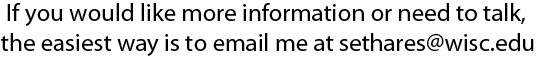# Weak Convergence and Local Stability Properties of Fixed Step Size Recursive Algorithm#### by J.A. Bucklew, T.G. Kurtz, W.A. Sethares

A recursive equation which subsumes several common adaptive filtering algorithms is analyzed for general stochastic inputs and disturbances by relating the motion of the parameter estimate errors to the behavior of an unforced deterministic ordinary differential equation (ODE). Local stability of the ODE implies long term stability of the algorithm while instability of the differential equation implies nonconvergence of the parameter estimates. The analysis does not require continuity of the update equation, and the asymptotic distribution of the parameter trajectories for all stable cases (under some mild conditions) is shown to be an Ornstein - Uhlenbeck process.

The ODE's describing the motion of several common adaptive filters are examined in some simple settings, including the Least Mean Square (LMS) algorithm and all three of its signed variants (the signed regressor, the signed error, and the sign-sign algorithms). Stability and instability results are presented in terms of the eigenvalues of a correlation-like matrix. This generalizes known results for LMS, signed regressor and signed error LMS, and gives new stability criteria for the sign-sign algorithm. The ability of the algorithms to track moving parameterizations can be analyzed in a similar manner, by relating the time varying system to a forced ODE. The asymptotic distribution about the forced ODE is again (under similar conditions) an Ornstein-Uhlenbeck process, whose properties can be described in a

This study of the behavior of adaptive algorithms first appeared in the IEEE Transactions on Information Theory in May 1993. You can download a .pdf version of this paper.To get to my homepage, click here.